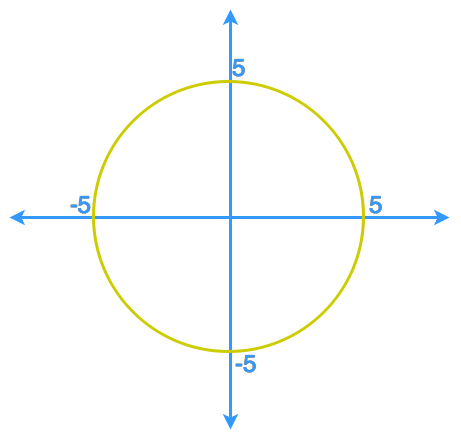# Implicit differentiation#### Everything You Need in One Place

Homework problems? Exam preparation? Trying to grasp a concept or just brushing up the basics? Our extensive help & practice library have got you covered.#### Learn and Practice With Ease

Our proven video lessons ease you through problems quickly, and you get tonnes of friendly practice on questions that trip students up on tests and finals.#### Instant and Unlimited Help

Our personalized learning platform enables you to instantly find the exact walkthrough to your specific type of question. Activate unlimited help now!

0/1
##### Intros
###### Lessons
1. Explicit Functions VS. Implicit Functions
0/2
##### Examples
###### Lessons
1. The graph shows a circle centred at the origin with a radius of 5.a) Define the circle implicitly by a relation between x and y .
b) Define the circle by expressing y explicitly in terms of x .
c) Use the method of "explicit differentiation" to find the slope of the tangent line to the circle at the point (4, -3).
d) Use the method of "implicit differentiation" to find the slope of the tangent line to the circle at the point (4, -3).
1. $3{y^4} + 5{x^2}{y^3} - {x^6} = 2x - 9y + 1$
Use implicit differentiation to find: $\frac{{{d}y}}{{{d}x}}$
###### Topic Notes
So far, we have always tried to configure a relation to an explicit function in the form of y = f(x) before finding the derivative of the relation, but what if this is impossible to do so? In this section, we will first learn to identify the difference between explicit functions and implicit functions. Then we will learn how to differentiate a relation with a mix of variables x and y using the method called Implicit Differentiation.